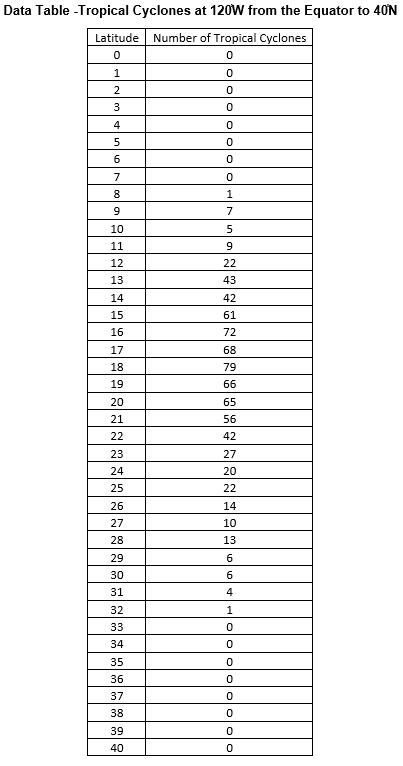## Tropical Cyclone Counts Create Histogram

The purpose of this lesson is for students to create a histogram using tropical cyclone data. This is part of the Tropical Cyclone Counts Graphing Bundle and can be completed independently or with the other activities in the bundle.

### Mini Lesson

#### Review histograms briefly.

A histogram is a type of graph which shows variability, or how spread out the data are, within a group.

Present examples of the types of questions that might be answered with a histogram:

1. How many smartphones were sold during the holidays in the different states in the U.S.?
2. What is the range of salaries for teachers in my state?
3. How many chocolate chips are in each of 100 bags of chocolate chips?

What do these questions have in common?

#### Plan your Histogram

Review the questions below with your students prior to distributing the materials in the next section.

1. Use the data table provided to make a frequency table with bins that are 5 units wide.

2. What is the x axis?

3. How many squares of the graph paper will you use for each bin?

4. What is the y axis?

5. How much will each square of the graph paper represent?

6. How many squares will you need to graph the tallest bar?

7. What title will you use?Create the Histogram Using the Answers to the Planning Questions

1. Draw the axes using the graph paper provided.

2. Label the axes.

3. Add the title.

4. Using the frequency table, draw and shade the bars for the histogram.